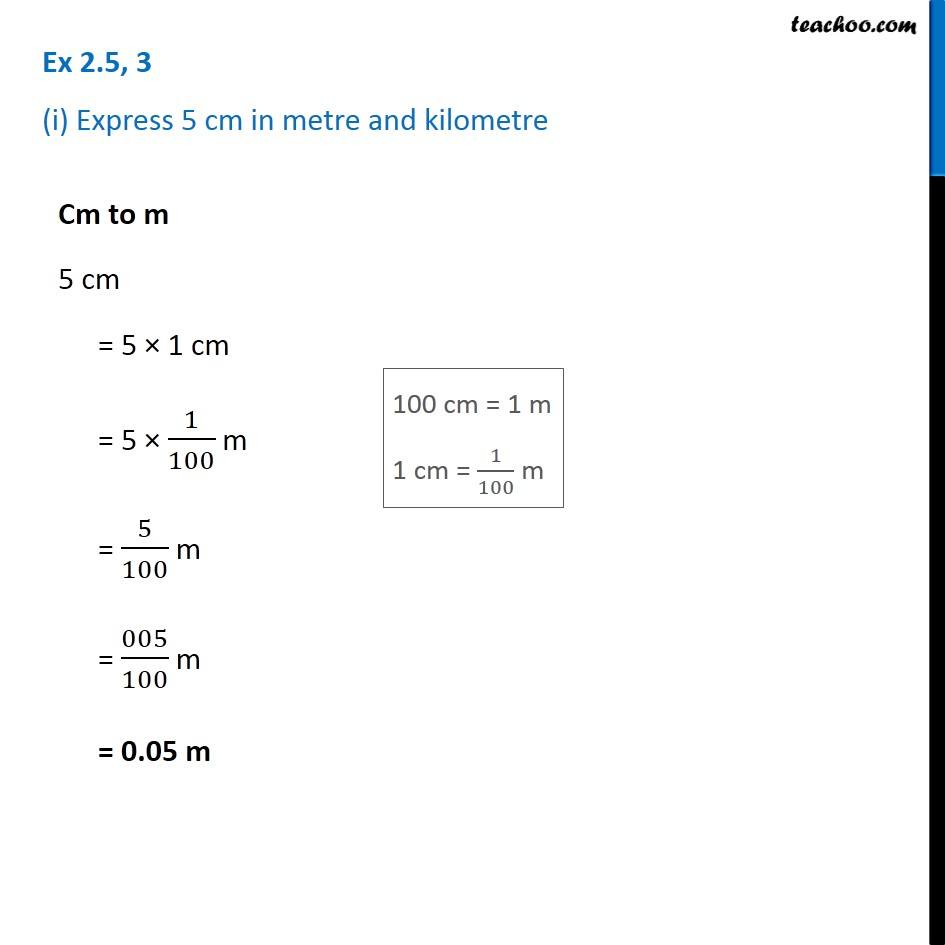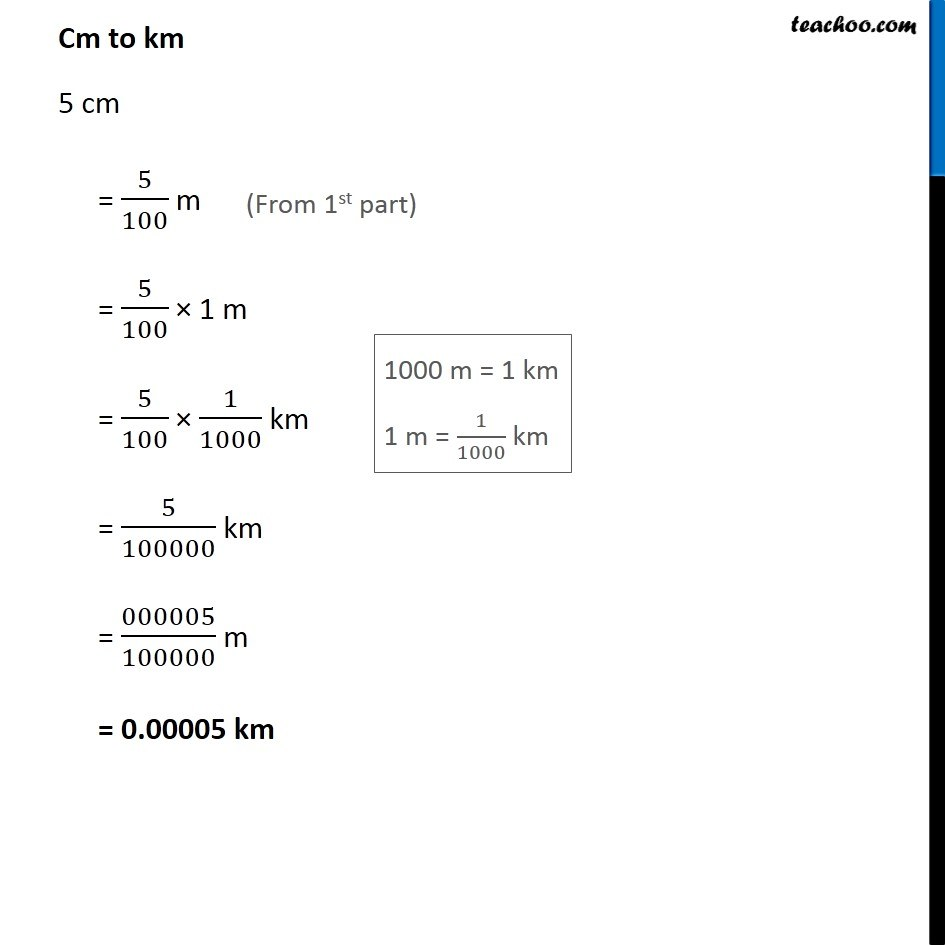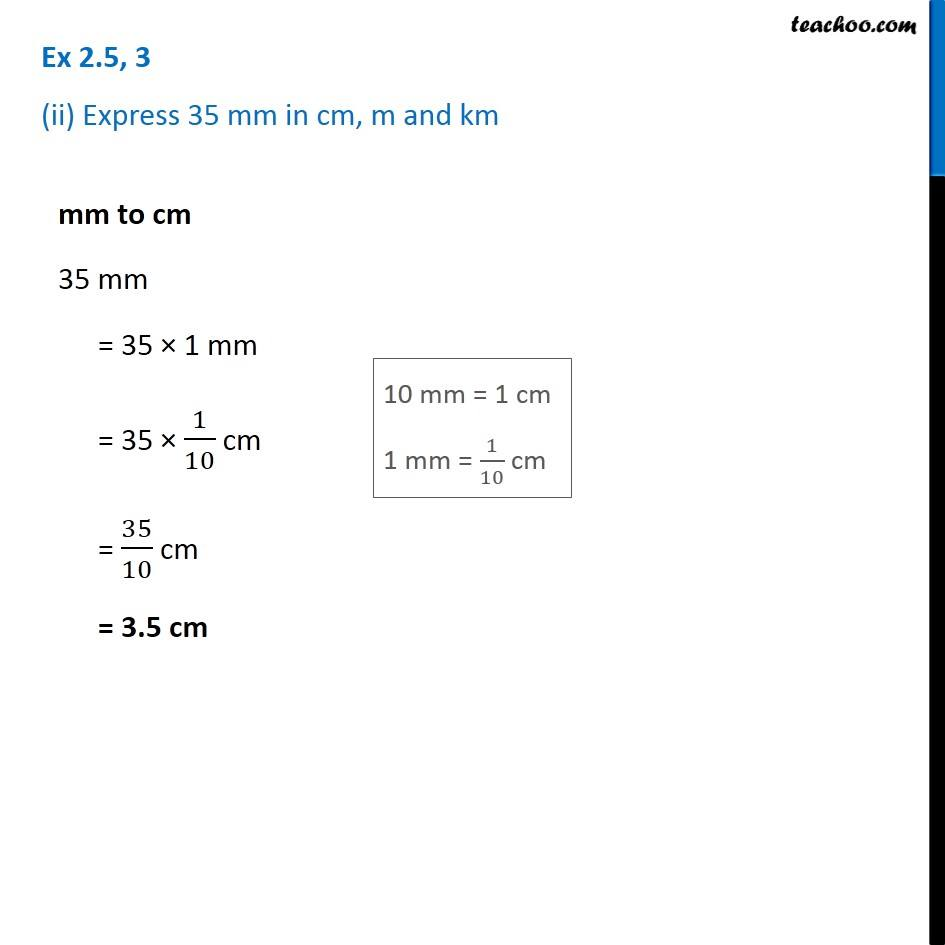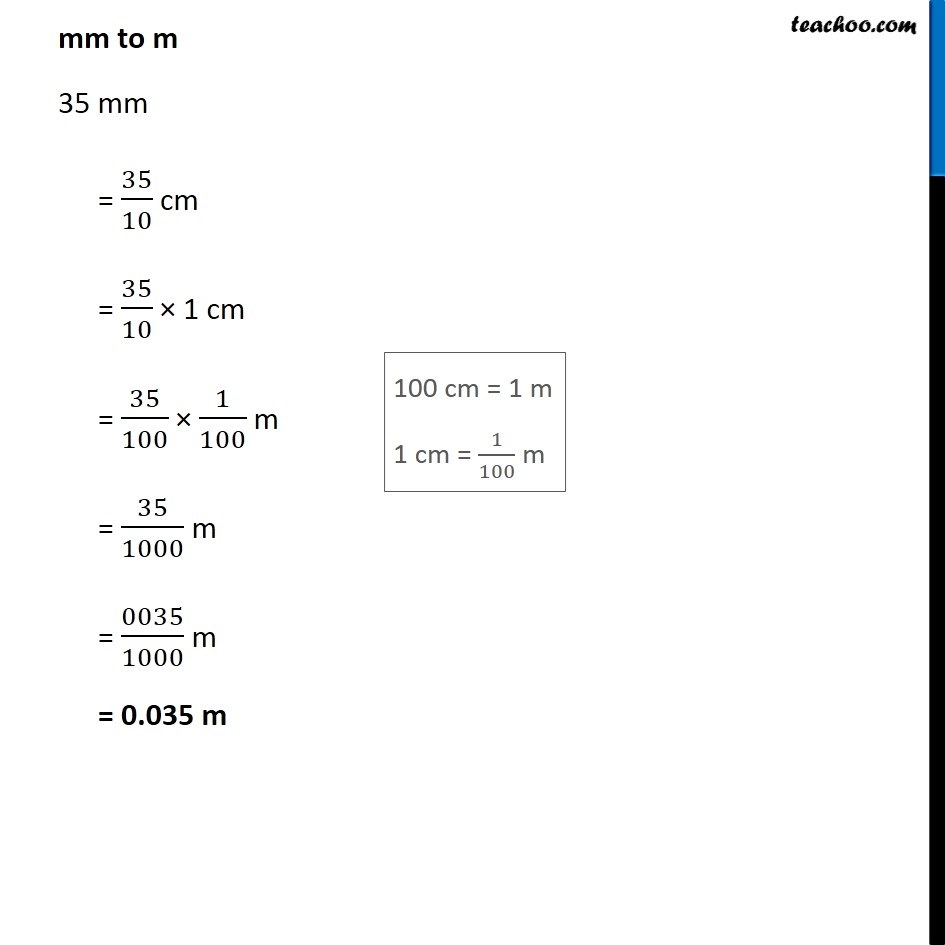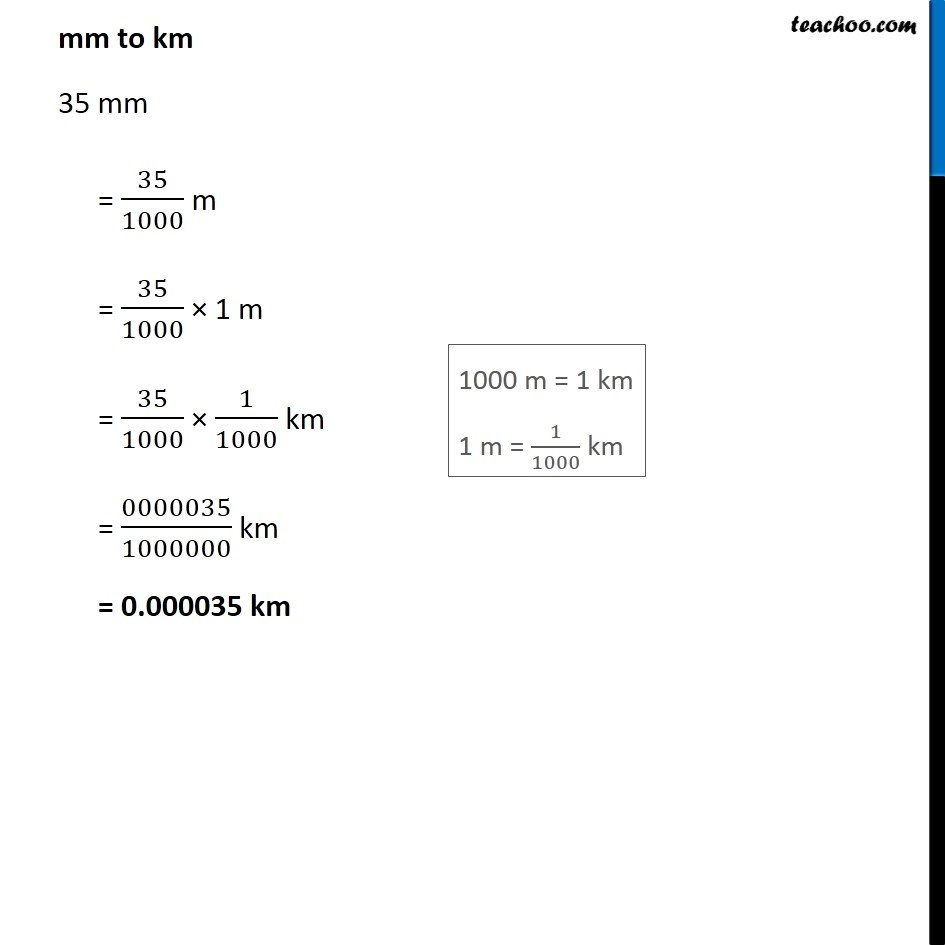Subscribe to our Youtube Channel - https://you.tube/teachoo

1. Chapter 2 Class 7 Fractions and Decimals
2. Serial order wise
3. Ex 2.5

Transcript

Ex 2.5, 3 (i) Express 5 cm in metre and kilometreCm to m 5 cm = 5 × 1 cm = 5 × 1/100 m = 5/100 m = 005/100 m = 0.05 m Cm to km 5 cm = 5/100 m = 5/100 × 1 m = 5/100 × 1/1000 km = 5/100000 km = 000005/100000 m = 0.00005 km Ex 2.5, 3 (ii) Express 35 mm in cm, m and km mm to cm 35 mm = 35 × 1 mm = 35 × 1/10 cm = 35/10 cm = 3.5 cm mm to m 35 mm = 35/10 cm = 35/10 × 1 cm = 35/100 × 1/100 m = 35/1000 m = 0035/1000 m = 0.035 m mm to km 35 mm = 35/1000 m = 35/1000 × 1 m = 35/1000 × 1/1000 km = 0000035/1000000 km = 0.000035 km

Ex 2.5